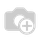# Date Modification

How to increase or decrease the current date (code in python file) ?

1 CommentPlease make the question more clear and detailed. In the case date, there is a large area to explain, so more specific, and exact need too. Thanks

You could use TIMEDELTA to increase or decrease date value.

from datetime import datetime, timedelta
print (datetime.today() + timedelta(days=(1))).strftime('%Y-%m-%d')    // for next day
print (datetime.today() + timedelta(days=(-1))).strftime('%Y-%m-%d')    // for previous day

1 CommentIt is better to use underscore in filename than any other special character since it widely accepted Therefore constructing file name as below:

``````from elasticsearch import Elasticsearch
import csv

es = Elasticsearch(["9200"])

# Replace the following Query with your own Elastic Search Query
res = es.search(index="search", body=
{
"_source": ["DTDT", "TRDT", "SPLE", "RPLE"],
"query": {
"bool": {
"should": [
{"wildcard": {"CN": "TEST1"}}

]
}
}
}, size=10)

from datetime import datetime
import os

file_path = <PASS YOUR FILE HERE>

csv_file = 'myfile_' + str(datetime.now().strftime('%Y_%m_%d_%H_%M_%S')) + '.csv'

csv_file_full = os.path.join(file_path, os.sep, csv_file)

header_names = { 'DTDT': 'DATE', 'TRDT': 'TIME', ...}

with open(csv_file_full, 'w') as f:  # Just use 'w' mode in 3.x